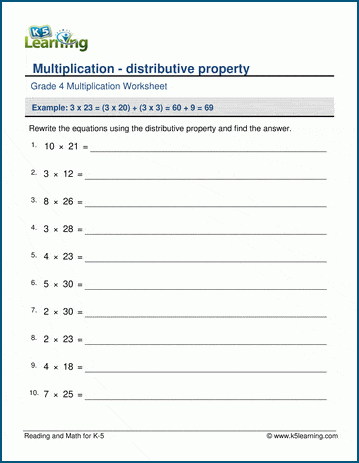# Distributive Property of Multiplication

The distributive property states that an expression which is given in form of A (B + C) can be solved as A × (B + C) = AB + AC. How would you explain this to your child?

First, you’ll want to tell them what distributive property in math is.

## What is distributive property?

The distributive property is a math rule that helps us when we need to multiply a number by a group of numbers added together. It makes things easier and helps us do math faster.

The best way to explain this is in an example.

## Distributive property example

Imagine you have this math problem:

3 x (4 + 2)

To use the distributive property, you do this:

First, you multiply 3 by 4: 3 x 4 = 12

Then, you multiply 3 by 2: 3 x 2 = 6

Now, you have two answers: 12 and 6.

Finally, you add those two answers together: 12 + 6 = 18

So, 3 x (4 + 2) equals 18.

The distributive property is like breaking a big problem into smaller parts, multiplying each part separately, and then adding those parts back together. It helps make multiplication easier!

## Worksheets to practice distributive property

In these worksheets, students practice using distributive property to multiply 1 by 2 digit numbers.Become a Member

This content is available to members only.